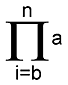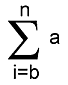### 6.1.6  User-Defined Functions

Some objects allow you to specify functions that will be evaluated while rendering to determine the surface of these objects. In this respect functions are quite different to macros, which are evaluated at parse time but do not otherwise affect rendering. Additionally you may call these functions anywhere a Float Function is allowed, even during parsing. The syntax is identical to Float Expressions, however, only float functions that apply to float values may be used. Excluded are for example `strlen` or `vlength`. You find a full list of supported float functions in the syntax definition below.

```FLOAT:
LOGIC_AND [OR LOGIC_AND]
OR:
|
LOGIC_AND:
REL_TERM [AND REL_TERM]
AND:
&
REL_TERM:
TERM [REL_OPERATOR TERM]
REL_OPERATOR:
< | <= | >= | > | = | !=
TERM:
FACTOR [SIGN FACTOR]
SIGN:
+ | -
FACTOR:
MOD_EXPRESSION [MULT MOD_EXPRESSION]
MULT:
* | /
EXPRESSION:
FLOAT_LITERAL         |
FLOAT_IDENTIFIER      |
FLOAT_FUNCTION        |
FLOAT_BUILT-IN_IDENT  |
FUNCTION_IDENTIFIER   |
( FLOAT )             |
IDENTIFIER            |
SIGN EXPRESSION
FLOAT_FUNCTION:
abs( FLOAT ) | acos( FLOAT ) | acosh( FLOAT ) | asin( FLOAT ) |
asinh( FLOAT ) | atan( FLOAT) | atanh( FLOAT) |
atan2( FLOAT , FLOAT ) | ceil( FLOAT ) | cos( FLOAT ) |
cosh( FLOAT ) | degrees( FLOAT ) | exp( FLOAT ) |
floor( FLOAT ) | int( FLOAT ) | ln (Float) | log( FLOAT ) |
max( FLOAT , FLOAT, ... ) | min( FLOAT , FLOAT, ... ) |
mod( FLOAT , FLOAT ) | pow( FLOAT , FLOAT ) |
radians( FLOAT ) | sin( FLOAT ) | sinh( FLOAT ) |
sqrt( FLOAT ) | tan( FLOAT ) | tanh( FLOAT ) |
select( FLOAT , FLOAT , FLOAT [, FLOAT] )
FUNCTION_IDENTIFIER:
#local FUNCTION_IDENTIFIER = function { FLOAT }               |
#declare FUNCTION_IDENTIFIER = function { FLOAT }             |
#local FUNCTION_IDENTIFIER = function(IDENT_LIST) { FLOAT }   |
#declare FUNCTION_IDENTIFIER = function(IDENT_LIST) { FLOAT } |
#local FUNCTION_IDENTIFIER = function{SPECIAL_FLOAT_FUNCTION} |
#local VECTOR_IDENTIFIER = function{SPECIAL_VECTOR_FUNCTION}  |
#local COLOR_IDENTIFIER = function { SPECIAL_COLOR_FUNCTION } |
IDENT_LIST:
IDENT_ITEM [, IDENT_LIST]
IDENT_ITEM:
x | y | z | u | v | IDENTIFIER
(Note: x = u and y = v)
SPECIAL_FLOAT_FUNCTION:
pattern { PATTERN_BLOCK }
SPECIAL_VECTOR_FUNCTION:
TRANSFORMATION_BLOCK | SPLINE
SPECIAL_COLOR_FUNCTION:
PIGMENT
PATTERN_BLOCK:
PATTERN
```

Note: Only the above mentioned items can be used in user-defined functions. For example the rand() function is not available.

All of the above mentioned float functions are described in the section Float Functions.

#### 6.1.6.1  Sum and Product functions

`prod(i, b, n, a)` The product function.product function

`sum(i, b, n, a)` The sum function.sum function

For both `prod` and `sum`: `i` is any variable name and `a` is any expression, usually depending on `i`. `b` and `n` are also any expression.
Example:

```  #declare factorial = function(C) { prod(i, 1, C, i) }
#declare A = factorial(5);
```

The first parameter is the name of the iteration variable. The second is the initial value expression and the third is the final value expression. Those may not depend on the iteration variable but the iteration variable may still be used inside those two expressions (because it happens to already have been defined) but its value is undefined. The last expression is the actual expression which will be iterated through. It may use any variable in scope.

The scope of an iteration variable is the sequence operation function. That is, a iteration variable is only defined when used inside the `sum/prod` function. Of course `sum/prod` functions may be nested. However, there is one limit of a maximum of 56 local variable defined simultaneously, which essentially means that in any combination `sum/prod` functions cannot be nested deeper than 56 levels.

The iteration variable is incremented by one for each step, but its initial and final value may be any value. The iteration will be continued as long as the iteration value is less or equal to the final value.

Note: because the iteration value is a floating-point variable, adding one will add a certain bias in a long iterations and thus the floating-point precision will be an issue in such a case and needs to be considered by allowing a reasonable error for the final value!

If the expression to be added has a negative sign it will of course in effect be substracted. Thus changing the sign will allow to generate negative values in the sum function. Equally multiplying by `1/expression` effectively creates a division when used in the prod function.

Obviously to work in the first place the initial value of the result is the neutral element of the operation. That is, a sum calculation starts with `0` and a product calculation starts with `1` just like it is assumed in the sum and product functions in 'regular' math.

It should be noted that mathematically either sum or product are redundant because:

```    log10(prod(i, b, n, a)) = sum(i, b, n, log10(a))
```
which implies a sum can be represented as a product and vice versa, observing the usual mathematical constraints of logarithms, of course. However, as logarithms and their inverse (powers) are slow to compute both are provided...

#### 6.1.6.2  Functions and Macros

You can use macros in functions, but the macros will be called only once when the function is defined, not every time the function is called. You cannot pass function variables to the macros.

You can pass functions to macros, how to do this is best explained by an example:

```  #macro Foo( Bar, X )
#declare Y = Bar(X);
#declare Z = Bar(Y);
#end

#declare FUNC=function(n){n+2}

Foo(FUNC, 1)

#debug str(Y,5,5)
#debug "\n"
#debug str(Z,5,5)
#debug "\n"
```

#### 6.1.6.3  Declaring User-Defined Float Functions

You declare a user defined function using the `#declare` or `#local` directives. By default a function takes three parameters and you do not have to explicitly specify the parameter names. The default three parameters are `x`, `y` and `z`. For example:

``` #declare foo = function { x + y * z }
```

If you need fewer or more parameters you have to explicitly specify the parameter list.

Note: `x` and `u` as well as `y` and `v` are equivalent so you may not specify both parameter names. You may not specify two or more parameters with the same name either. Doing so may result in a parse error or undefined function results.

The following are valid functions with parameters:

``` #declare foo2 = function(x, y, z) { x + y * z }
#declare foo3 = function(k1, k2, z, y) { x + y * z + k1 * y + k2 }
#declare foo4 = function(h) { h * h + h }
#declare foo4 = function(u, v) { x + y * v } //=u + v*v
#declare foo4 = function(x, v, z) { u + y * v + z } //=x + v*v + z
```

Limits:

• The minimum number of parameters per function is 1.
• The maximum number of allowed parameters per function is 56.
• The maximum number of `function` blocks per scene is 1048575.
• The maximum number of operators per function is about 200000. Individual limits will be different depending on the types of operators used in the function.
• The maximum depth for nesting functions is 1024.
• The maximum number of constants in all functions 1048575.

Note: Redeclaring functions, directly, is not allowed. The way to do this is to `undef` it first.

There is one special float function type. You may declare a `pattern` function.

Note: the syntax is identical to that of patterns, however, you may not specify colors. Its result is always a float and not a color vector, as returned by a function containing a pigment.

``` #declare foo = function {
pattern {
checker
}
}
```

Note: the number of parameters of special function types is determined automatically, so you do not need to specify parameter names.

#### 6.1.6.4  Declaring User-Defined Vector Functions

Right now you may only declare vector functions using one of the special function types. Supported types are `transform` and `spline` functions. For example:

``` #declare foo = function {
transform {
rotate <90, 0, 0>
scale 4
}
}

#declare myvector = foo(4, 3, 7);

#declare foo2 = function {
spline {
linear_spline
0.0, <0,0,0>
0.5, <1,0,0>
1.0, <0,0,0>
}
}

#declare myvector2 = foo2(0.7);
```

Function splines take the vector size into account. That is, a function containing a spline with five components will also return a five component vector (aka a color), a function containing a spline with two components will only return a two component vector and so on.

Note: the number of parameters of special function types is determined automatically, so you do not need to specify parameter names.

#### 6.1.6.5  Declaring User-Defined Color Functions

Right now you may only declare color functions using one of the special function types. The only supported type is the `pigment` function. You may use every valid `pigment`. This is a very simple example:

``` #declare foo = function {
pigment {
color red 1
}
}

#declare Vec = foo(1,2,3)
```

An example using a pattern:

``` #declare foo = function {
pigment {
crackle
color_map {
[0.3, color Red]
[1.0, color Blue]
}
}
}

#declare Val = foo(2,3,4).gray
```

Note: the number of parameters of special function types is determined automatically, so you do not need to specify parameter names.

#### 6.1.6.6  Internal Pre-Defined Functions

Several functions are pre-defined. These internal functions can be accessed through the "functions.inc", so it should be included in your scene.
The number of required parameters and what they control are also given in the include file, but the "functions.inc" chapter in the "Standard Include File" section gives more information.